Next: 6.2.4 Extended Poisson-Boltzmann Model Up: 6.2 Modeling BioFETs Previous: 6.2.2 Poisson-Boltzmann Model with

## 6.2.3 Poisson-Boltzmann Model with Homogenized Interface Conditions

A similar but somewhat refined model was derived in [219,220,221]. The authors solved the multi-scale problem by exchanging the fast varying charge distribution at the surface (e.g. Proteins or DNA fragments scattered over the functionalized surface) by two interface conditions. These interface conditions describe the effects of the charge and the dipole moment of the biofunctionalized layer, including the charged macromolecules: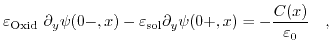(6.3)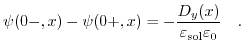(6.4)

Here,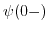describes the potential at the dielectric-electrolyte interface from the oxide, while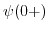denotes the interface potential from the solute. The first equation describes the jump in the field, while the second introduces a dipole moment which causes a shift of the potential.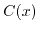is the averaged (homogenized) charge density at the dielectric-electrolyte interface and can either be determined by experimental data or derived from first principle calculations via a data set from a protein data bank .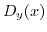is the averaged dipole moment density and has to be gained from first principle calculations. For instance, the adaptive Poisson-Boltzmann solver (APBS) [222,223,224] allows to assign partial charges to every atom belonging to the desired macromolecule, and therefore the calculation of the overall charge and in conjunction with the relative distances between the atoms also the dipole moment of the molecule. This charge and dipole moment can be linked to the mean charge and mean dipole moment assuming an average distance between the macromolecules.Next: 6.2.4 Extended Poisson-Boltzmann Model Up: 6.2 Modeling BioFETs Previous: 6.2.2 Poisson-Boltzmann Model with

T. Windbacher: Engineering Gate Stacks for Field-Effect Transistors